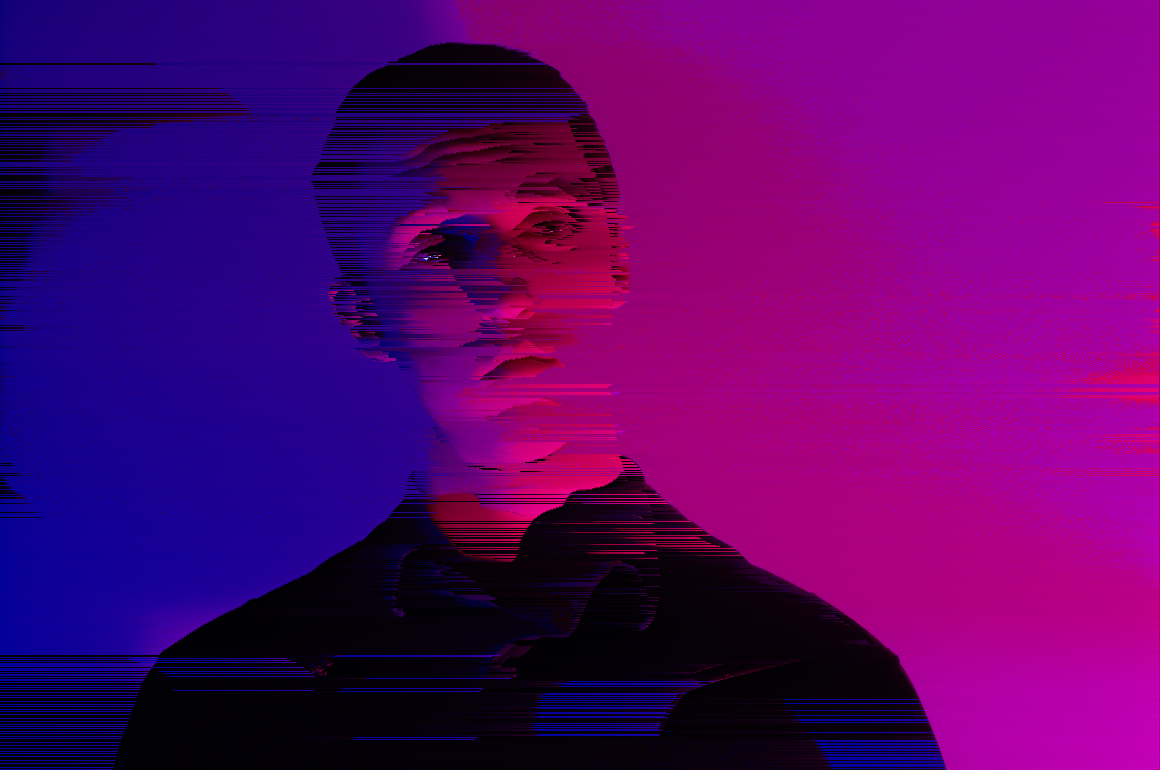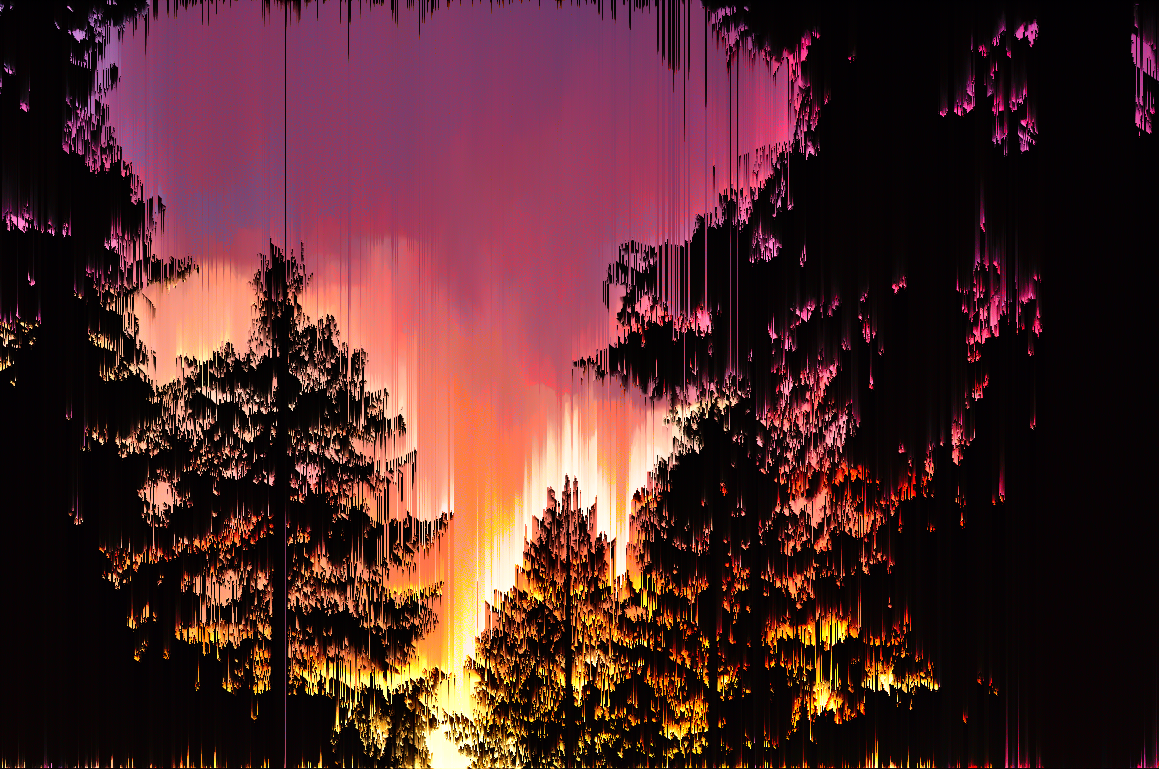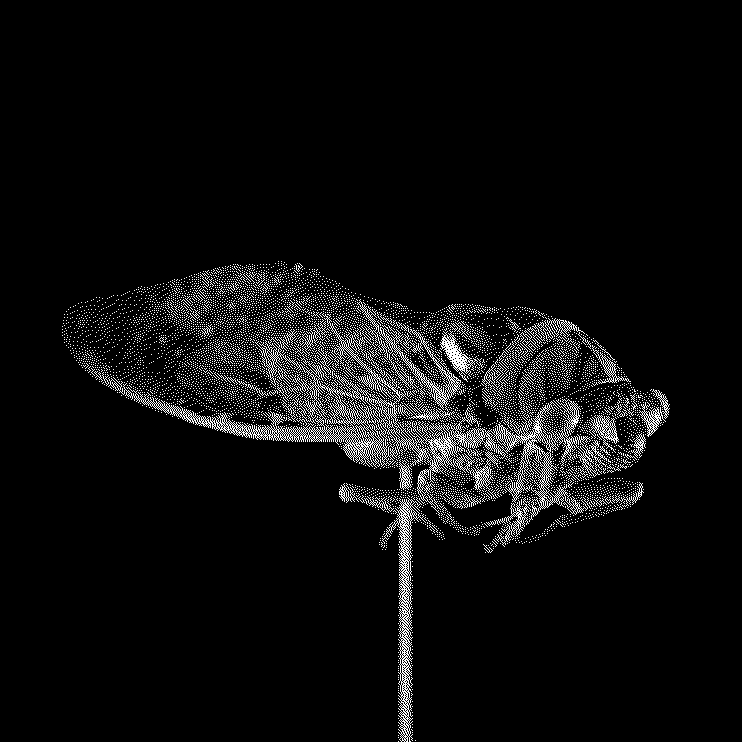Getting GlitchyComputers are these machines that we have engineered to be totally deterministic. No matter how many times a computer is provided with with some input, it should always give the same output. This is why glitch art is so fascinating - it happens when computers dont work the way they are supposed to. Of course computers crap out all the time, but that is usually due to poor programming for end-user applications. Actual data corruption is much rarer, and can both wreak havoc and be utterly entrancing.

The thumbnail for this particular post is not a true glitch. Rather it is merely a simulation - an attempt at recreating the effects of data corruption. This instance in paricular uses a technique called pixel sorting. I am not the first person to have done this - not by far. However, I pride myself on writing original code to accomplish this task based only on example images that I have seen on the internet.

My first observation on pixel sorting was that while pixels are sorted along a single axis, they are not sorted across the full length of that axis. If they were, the image would be a fairly boring gradient. Instead, the sorting seems to respect an image’s features in some way. I decided to use edge detection to maintain the “shape” of the image and delimit the areas in which pixels may be sorted.

Edge Detection

After a bit of research, the Canny Edge Detector seemed to be the way to go. It produces as its output a very clean 1-bit image. That is to say, it does a good job of ignoring noise, and gives a definitive single-pixel wide edge.

The wikipedia article does a fairly good job of describing the algorithm. The main part I had to implement on my own was the hysteresis function, which determines whether or not an edge ought to be included in the final output.

 1 2 3 4 5 6 7 8 9 10 11 12 13 14 15 16 17 18 19 20 21 22 23 24 25 26 27 28 29 30 31 32 33 34 35 36 37 38 39 void chain(image& img, int r, int c, bool** visited){ if(visited[r][c]) return; //already been here, don't bother visited[r][c] = true; //we can only get here from a strong pixel, so we can make this one strong. img[r][c] = pixel(1.0); //look at the 3x3 grid around [r][c] for(int i = r - 1; i <= r + 1; i++){ for(int j = c - 1; j <=c + 1; j++){ //if the pixel is out of bounds, ignore it. if(i < 0 || j < 0 || i >= img.r() || j >= img.c()) continue; //if the next pixel is strong, or is a candidate, add it to the chain. if(!visited[i][j] && img[i][j].y >= 0.5) chain(img, i, j, visited); } } return; } void hysteresis(image& img, std::vector slist){ bool** visited = new bool*[img.r()]; for(int i = 0; i < img.r(); i++){ visited[i] = new bool[img.c()]; for(int j = 0; j < img.c(); j++){ visited[i][j] = false; } } for(int i = 0; i < slist.size(); i++){ //start a chain IFF the pixel is strong. if(img[slist[i].row][slist[i].col].y == 1.0){ chain(img, slist[i].row, slist[i].col, visited); } } for(int i = 0; i < img.r(); i++){ delete[] visited[i]; } delete[] visited; //remove any unconnected weak edges threshold(img, 1.0); }

The hysteresis() function accepts an image of the preliminary edges in which an edge is either strong, with a value of 1, or weak with a value of 0.5. All other pixels are set to 0. The parameter slist is simply a list of all the pixels that were determined to be a strong edge. This prevents scanning over the entire image again.

The chain() function is called by the hysteresis() function on all strong edges. It then looks at the 8 pixels around the one given. If it finds a weak pixel, since it is bordering a strong pixel it will be set to a strong pixel. chain() is then called recursively on the newly set pixel. In this way, we move along an edge, creating a “chain” of strong pixels until the edge ends. Once the hysteresis() function returns, the image only consists of strong edges.

Now that we have well-defined edges, we can sort pixels by brigtness. In the original image, we take the top row of pixels and starting from the left, scan until we hit either a strong edge or the end of the row. This section of pixels is then sorted. We then start from where we last stopped and repeat until the whole row is finished.

This simple process can create some rather stunning images:Other Fun

Once I had the program working, I had the backbone for a rather nice general image processing program. Therefore, I decided to take a stab at some dithering, and the code amounted to an impressive 18 lines:

 1 2 3 4 5 6 7 8 9 10 11 12 13 14 15 16 17 18 19 //Floyd-Steinberg void dither(image& img){ pixel oldpixel; pixel newpixel; double q_error; for(int i = 0; i < img.r(); i++){ for(int j = 0; j < img.c(); j++){ oldpixel = img[i][j]; newpixel = pixel(oldpixel.y > 0.5); img[i][j] = newpixel; q_error = oldpixel.y - newpixel.y; if (j < img.c() - 1) img[i ][j+1].y += q_error * 7.0 / 16.0; if (j > 0 && i < img.r() - 1) img[i+1][j-1].y += q_error * 3.0 / 16.0; if (i < img.r() - 1) img[i+1][j ].y += q_error * 5.0 / 16.0; if (iI personally find dithered images to be rather pleasing to look at. (Scaling ruins the effect. Click in the image for a direct link and make sure it is at 100% zoom exactly)